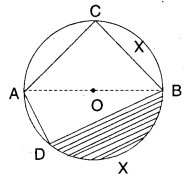AP State Syllabus AP Board 9th Class Maths Solutions Chapter 12 Circles Ex 12.1 Textbook Questions and Answers.

## AP State Syllabus 9th Class Maths Solutions 12th Lesson Circles Exercise 12.1Question 1.
Name the following from the given figure where ’O’ is the centre of the circle.
i) $$\overline{\mathbf{A O}}$$
ii) $$\overline{\mathbf{A B}}$$
iii) $$\widehat{\mathrm{BC}}$$
iv) $$\overline{\mathbf{A C}}$$
v) $$\widehat{\mathrm{DCB}}$$
vi) $$\widehat{\mathrm{ACB }}$$
vii) $$\overline{\mathbf{A D}}$$Solution:
i) $$\overline{\mathbf{A O}}$$ – radius
ii) $$\overline{\mathbf{A B}}$$ – diameter
iii) $$\widehat{\mathrm{BC}}$$ – minor arc
iv) $$\overline{\mathbf{A C}}$$ – chord
v) $$\widehat{\mathrm{DCB}}$$ – major arc
vi) $$\widehat{\mathrm{ACB }}$$ – semi circle
vii) $$\overline{\mathbf{A D}}$$ – chord
viii) Shaded region – Minor segmentQuestion 2.
State true or false.
i) A circle divides the plane on which it lies into three parts. ( )
ii) The area enclosed by a chord and the minor arc is minor segment. ( )
iii) The area enclosed by a chord and the major arc is major segment. ( )
iv) A diameter divides the circle into two unequal parts. ( )
v) A sector is the area enclosed by two radii and a chord. ( )
vi) The longest of all chords of a circle is called a diameter. ( )
vii) The mid point of any diameter of a circle is the centre. ( )
Solution:
i) True
ii) True
iii) True
iv) False
v) False
vi)True
vii) True